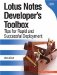# General Syntax Rules

The Formula Language syntax rules are quite simple. All formulas must adhere to the following five rules.

 Rule 1. A semicolon must separate all formulas that contain more than one statement. It's important to understand that multiple statements can reside on a single line, provided that a semicolon delineates each statement. For example, the following are valid multi-statement formulas. Example 1   ```DEFAULT status := "New Request"; status ``` Example 2   ```DEFAULT status := "New Request"; status ``` Rule 2. At least one space must follow all reserved keywords. Otherwise, any number of spacesfrom none to manymay be used to separate operators, punctuation, and values. For example, the following are valid and equivalent statements (with regard to spacing):   ```output := "Today's date is: " + @Today; output:="Today's date is:" + @Today; FIELD output:= "Today's date is: " + @Today; ``` Rule 3. Two values, variables, or strings must be separated by at least one operator. For example, the following are valid statements. In the first example, the plus sign is used to sum the two numbers and divide the result by 2. In the second example, the plus sign is used to concatenate two values together. Finally, the third example shows three @Functions separated by the plus operator.   ```output := (100 + 200)/2; output := "The sum of the two numbers is: " + total; output := @Month+@Day+@Year; ``` Rule 4. You must use the := assignment operator to assign a value to a variable, whereas the equal sign = is used to compare two values when used in a conditional statement (such as the @IF, @WHILE, or @FOR functions).   ```myVariable := "This is a valid assignment"; ``` Rule 5. All formulas must contain a main expression that either produces a value or performs an action. For example, the following are valid main expressions. The following returns today's date.   ```@Today ``` The following checks if the value stored in the result variable is equal to one. If the condition is true, then the formula returns the text string "Yes". Otherwise, the formula is set to a text string "No".   ```@If (result = 1; "Yes"; "No") ```Lotus Notes Developers Toolbox: Tips for Rapid and Successful Deployment
ISBN: 0132214482
EAN: 2147483647
Year: N/A
Pages: 293
Authors: Mark Elliott